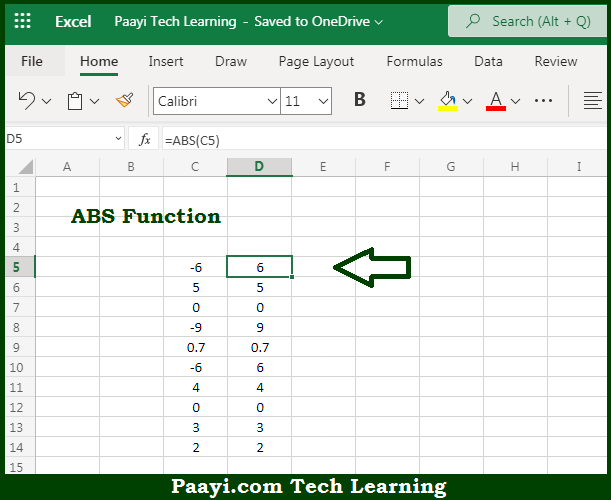# Learn How to Use Microsoft Excel ABS Function

Written by | 0 Comments | 557 Views

In this article, you will learn how to use the Microsoft Excel ABS function and its prime function in Microsoft Excel. You will also get to know the Microsoft Excel ABS function return value and syntax with the help of some examples.

Microsoft Excel ABS Function

The main function of the Microsoft Excel ABS function is to return the absolute value of a number. That means with the help of ABS function Negative numbers are converted to positive numbers, and positive numbers are unaffected. So, with the help of the Microsoft Excel ABS function, you can easily return the absolute value of the number.

Return Value of ABS Function

The return value will be the positive number.

Syntax of ABS Function

=ABS(number)

Where the argument:

• number: This is the number of which you want to get the absolute value.

How to Use Microsoft Excel ABS Function?Let us understand this ABS function with this example, ABS(-5) returns a value of 5 and ABS(5) returns a value of 5, the reason is ABS function returns a number's distance from zero. So, with the help of the Microsoft Excel ABS function, you can easily return the absolute value of the number.